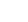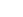" "

# What is the difference between GPA and CGPA?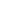Deepa Chandrashekar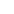Jan 9, 2023
Schools, Learning

Are you dreaming of pursuing your studies at top-notch Indian Universities? Wait, are you also planning to study abroad? Then, you must know that good grades are the best bet for you to get into any University, right?

Most universities pay keen attention to the GPA (Grade Point Average) and CGPA (Cumulative Grade Point Average) scores of the students to calculate, assess and grade the academic performance of the applying candidate.

Hence, you need to understand what is GPA and CGPA, the difference between GPA and CGPA, and the importance of GPA and CGPA to make your grades even better. So, let us begin.

## What is GPA?

GPA, a term that we have all been hearing since secondary school, stands for Grade Point Average. It refers to a student’s score on average. It could either be represented as a number or a letter.

## What is CGPA?

CGPA, another heard term, stands for Cumulative Grade Point Average. It indicates the overall academic performance of a student. It is generally

## Difference Between GPA and CGPA

Grade Point Average and Cumulative Grade Point Average are both used to compute the performance of a student in a course or a subject. GPA indicates the performance of the student in each semester whereas CGPA indicates the performance of the student in all the semesters in a year.

Hence, in order to score a high CGPA, a student must perform exceptionally well in all the semesters and work hard to earn a high GPA as well.

Formula to calculate GPA = Total grade points of all the courses or subjects in one semester / Total number of credits

Formula to calculate CGPA = Mean of the GPA scores of all the semesters / Total number of credits

## What is the formula to Calculate GPA?

The calculation of GPA is necessary to understand to get a better comprehension of the calculation of CGPA. So let’s begin. Generally, the grade represented as a letter stands for a number.

For example, grade A stands for a multiple of 4 and B stands for a multiple of 3. So if you score grade A, it means your GPA is 4. Similarly, if you score a grade of B, it means your GPA is 3. Furthermore, the scale shifts further as per the grading system.

Let’s say a student took 3 courses with 3 credits each and the student scores something like this-

● Course 1 - Grade A i.e, GPA score 4
● Course 2 - Grade B i.e, GPA score 3
● Course 3 - Grade C i.e, GPA score 2

Now, you need to multiply each GPA score with the number of credits mentioned i.e, 3.

● Course 1 - GPA Score 4 x 3 credits = 12
● Course 2 - GPA Score 3 x 3 credits = 9
● Course 3 - GPA Score 2 x 3 credits = 6

The total grade point is now 27. And the total number of credits is 9 which we derive from 3 credits for each subject i.e, 3+3+3 =9.

Now, we arrive at the formula, Total grade points divided by Total Credits is equal to Grade Point Average.

It means in this particular example, the GPA of the student is 3, which we derive from 27/9=3.

Hence, one must observe that the credits play an equally essential role in determining your grade point.

## What is the formula to Calculate CGPA?

Cumulative Grade Point Average or CGPA is obtained by calculating the mean GPA for every semester divided by the total number of credits.

Yes, it’s that easy! Once you have perfectly obtained your GPA score for each term, finding the CGPA can be easy.

## How to Calculate CGPA from GPA?

GPA plays an integral role in finding the CGPA. A student must gather the GPA score of all the semesters and find the mean of them altogether. Now, divide the obtained mean of the GPA by the total number of credits, and you shall get your CGPA score now.

## What is the Importance of GPA and CGPA?

GPA and CGPA are equally important to measure the performance of a student. GPA is the score that a student earns each semester whereas CGPA is the cumulative score of all the GPA scores earned in a year.

Hence, a student must pay keen attention to all the subjects and work really hard to obtain a good CGPA by the end of the year. In order to get into a reputed and successful university, attaining high scores in both GPA and CGPA are important.

### Let’s See What More Importance Do GPA And CGPA Hold

● GPA and CGPA are both needed for higher studies programs in India as well as abroad.
● A good GPA and CGPA scores can help you bag great scholarship programs.
● Students can apply for great placement opportunities with a high GPA and CGPA.
● All the universities ask for both GPA and CGPA scores (and not just CGPA).
● A student must maintain good GPA scores to get a high CGPA.

## Conclusion

It is time to metamorphose your dream into reality by focussing hard on your studies and setting your goals right. Understand the basic concepts between CGPA vs GPA. Ensure that you work hard to obtain a good GPA and CGPA scores.

It will help you apply for higher studies, scholarships, placements, and much more with your mere good performance. Therefore, attaining a deep comprehension of these terms will help you improvise immensely and be a bright student among the lot.

Global Indian International School is an international school in Bangalore that fosters students from around the world. The curriculum of school is designed in a way that students develop unique methods of studying and attain great GPA and CGPA scores.

## FAQs on GPA and CGPA

### Is GPA Higher than CGPA?

No. GPA is the average grade point earned by a student in a single semester. On the other hand, CGPA is the mean of all the GPA scores earned in all the semesters. Hence, it is the GPA that is higher.

### What is a Good CGPA?

A student earning more than 8 CGPA can be good for applying to the universities. It is the best shot to apply anywhere in the world in fact.

### Is a GPA of 3.14 Good?

3.14 is an average GPA score that we can consider to be good. But since it falls under Grade B, it can hamper your CGPA score in the end. Hence, GPA is the score of 4 is the ideal score that a student must obtain to perform well in CGPA.

### Do Universities Look at GPA or CGPA?

Most universities look for both GPA and CGPA scores to analyze the performance of the student.

### Can CGPA be in Odd Numbers?

No, CGPA cannot be in odd numbers.Deepa Chandrashekar

## More from same authorChat with counsellor

## Top Scholarships#### Global Future Ready Merit Scholarship

Eligibility:

90% & Above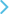#### Mahatma Gandhi Universal Values Merit-cum-Means Scholarship

Eligibility:

GROSS FAMILY INCOME : Below 8,00,000 INR to 25,00,000 INR#### Dr APJ Abdul Kalam Global Skills Scholarship

Eligibility:

Talent Based - Technology#### 9GEMS Holistic Development Scholarship

Eligibility:

Talent Based - 9 GEMS#### GIIS Global Sports Scholarship

Eligibility:

Sports Excellence#### Global Citizen Scholarship

Eligibility:

93.0% and Above